# Excel VLOOKUP not working - solutions for N/A, NAME and VALUE errors

The tutorial explains how you can quickly cope with VLOOKUP not working problems in Excel 2016, 2013, 2010, 2007 and 2003, troubleshoot and fix common errors and overcome VLOOKUP's limitations.

In the last few articles, we have explored different aspects of the Excel VLOOKUP function. If you have been following us closely, by now you should be an expert in this area : )

However, it's not without a reason that many Excel specialists consider VLOOKUP to be one of the most intricate Excel functions. It has a ton of limitations and specificities, which are the source of various problems and errors.In this article, you will find simple explanations of VLOOKUP's #N/A, #NAME and #VALUE error messages as well as solutions and fixes. We will start with the most frequent cases and most obvious reasons why vlookup is not working, so it might be a good idea to check out the below troubleshooting steps in order.

#### The tool that uses either VLOOKUP or INDEX/MATCH

##### Depending on your Excel dataset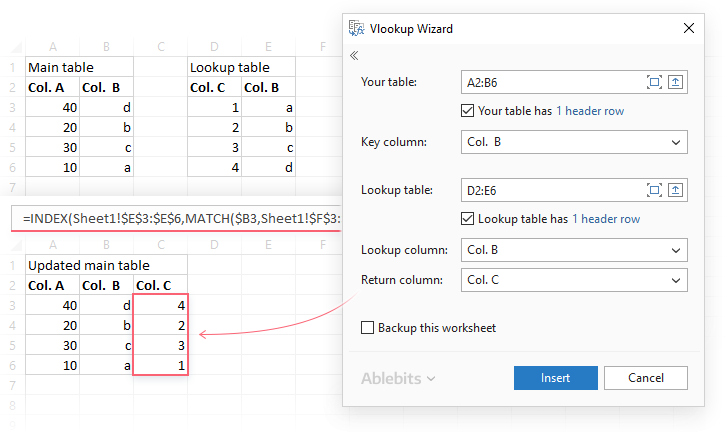Read more

#### This tool merges Excel tables and builds formulas for you

##### You just select your ranges and columns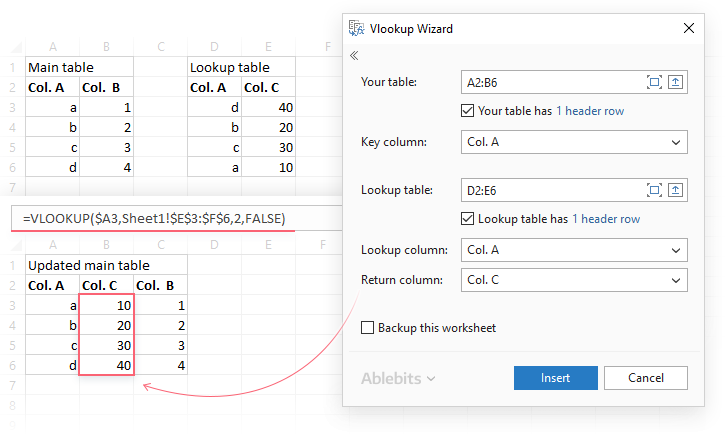Read more

#### Excel VLOOKUP Wizard

##### Easy way to build complex VLOOKUP formulas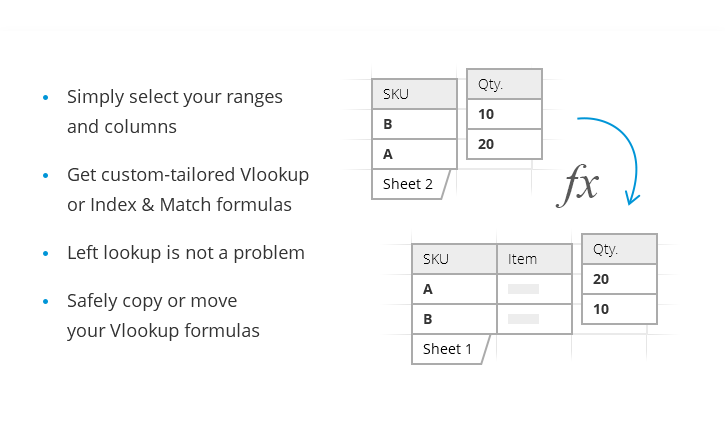Read more

## Fixing VLOOKUP N/A error in Excel

In Vlookup formulas, the #N/A error message (meaning "not available") is displayed when Excel cannot find a lookup value. There can be several reasons why that may happen.

#### 1. A typo or misprint in the lookup value

It's always a good idea to check the most obvious thing first : ) Misprints frequently occur when you are working with really large data sets consisting of thousands of rows, or when a lookup value is typed directly in the formula.

#### 2. #N/A in approximate match VLOOKUP

If you are using a formula with approximate match (range_lookup argument set to TRUE or omitted), your Vlookup formula might return the #N/A error in two cases:

• If the lookup value is smaller than the smallest value in the lookup array.
• If the lookup column is not sorted in ascending order.

#### 3. #N/A in exact match VLOOKUP

If you are searching with exact match (range_lookup argument set to FALSE) and the exact value is not found, the #N/A error is also returned. See more details on how to properly use exact and approximate match VLOOKUP formulas.

#### 4. The lookup column is not the leftmost column of the table array

As you probably know, one of the most significant limitations of Excel VLOOKUP is that it cannot look to its left, consequently your lookup column should always be the left-most column in the table array. In practice, we often forget about this and end up with VLOOKUP not working because of the N/A error.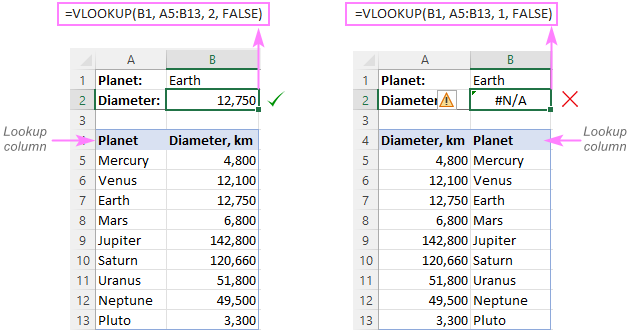Solution: If it is not possible to restructure your data so that the lookup column is the left-most column, you can use a combination of Excel's INDEX and MATCH functions, as a more versatile alternative to VLOOKUP. You will find the detailed info and a formula example in this tutorial - INDEX / MATCH formula to lookup values to left.

#### 5. Numbers are formatted as text

Another source N/A errors in VLOOKUP formulas is numbers being formatted as text, either in the main or lookup table.

This usually occurs when you import data from some external database or if you've typed an apostrophe before a number to indicate a leading zero.

The most obvious indications of numbers being formatted as text are shown in the screenshot below.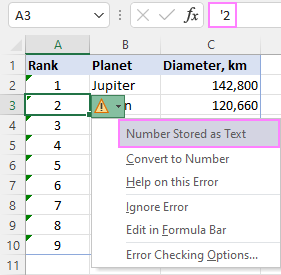The numbers can also be stored in the General format. In this case, there's only one noticeable sign - numbers get aligned to the left side of a cell, while numbers stored as numbers are aligned to the right by default.

Solution: If this is just a single number, simply click on the error icon and choose "Convert To Number" from the context menu.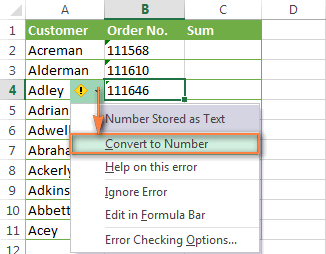If multiple numbers are affected, select them all, right-click the selection, then choose Format Cells > Number tab > Number and click OK.

#### 6. Excess leading or trailing spaces

This is the least obvious cause of the Vlookup N/A error because a human eye can hardly spot those extra spaces, especially when working with big tables where most of the entries are below the scroll.

Solution 1: Extra spaces are in the main table (with VLOOKUP formulas)

If excess spaces occur in your main table, you can ensure the correct work of your Vlookup formulas by wrapping the lookup_value argument with the TRIM function:

`=VLOOKUP(TRIM(\$F2),\$A\$2:\$C\$10,3,FALSE)`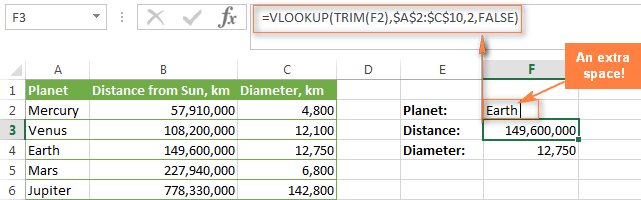Solution 2: Extra spaces are in the lookup table (lookup column)

If extra spaces occur in the lookup column, there is no easy way to avoid VLOOKUP #N/A errors. Instead of VLOOKUP, you can use an array formula with a combination of INDEX / MATCH and TRIM functions:

`=INDEX(\$C\$2:\$C\$10,MATCH(TRUE,TRIM(\$A\$2:\$A\$10)=TRIM(\$F\$2),0))`

Since this is an array formula, don't forget to press Ctrl + Shift + Enter rather than a usual Enter keystroke to properly complete it: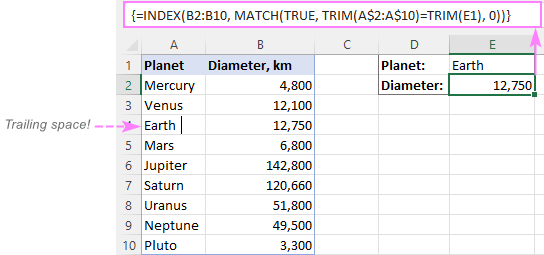For more information about using INDEX / MATCH in Excel, please check out this tutorial: INDEX & MATCH in Excel - a better alternative to VLOOKUP.

Tip. A quick alternative to complex INDEX / MATCH formulas is running the Trim Spaces for Excel add-in that will eliminate excess spaces both in the lookup and main tables in seconds, making your VLOOKUP formulas error-free. This is a free tool and you can find the download link on the above page.

## #VALUE error in VLOOKUP formulas

In general, Microsoft Excel displays the #VALUE! error if a value used in the formula is of a wrong data type. In respect to VLOOKUP, there are two common sources of the VALUE! error.

#### 1. Lookup value exceeds 255 characters

Please be aware that the VLOOKUP function cannot look up values containing 256 characters or more. If your lookup values exceed this limit, you will end up having the VALUE error: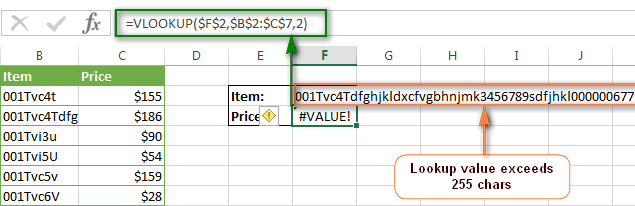Solution: Use an analogous INDEX /MATCH formula instead. In the above example, the following INDEX / MATCH function works perfectly:

`=INDEX(C2:C7,MATCH(TRUE,INDEX(B2:B7= F\$2,0),0))`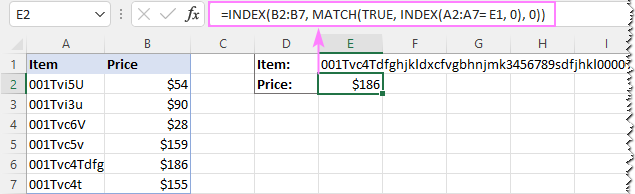#### 2. Full path to the lookup workbook is not supplied

If you are pulling data from another workbook, you have to include the full path to that file. More precisely, you have to enclose the workbook's name (including the extension) in square brackets [], and then specify the sheet's name followed by the exclamation mark. Also, you should have apostrophes around all this in case either a workbook or spreadsheet name contains spaces.

Here's the structure of the complete formula to do a vlookup from another workbook:

=VLOOKUP(lookup_value, '[workbook name]sheet name'!table_array, col_index_num, FALSE)

A real formula might look similar to this:

`=VLOOKUP(\$A\$2,'[New Prices.xls]Sheet1'!\$B:\$D,3,FALSE)`

The above formula will search for the value of cell A2 in column B of Sheet1 in the "New Prices" workbook, and return a matching value from column D.

If any element of the path is missing, your VLOOKUP formula won't work and return the #VALUE error (unless the lookup workbook is currently open).

For more info about VLOOKUP formulas referencing another Excel file, please check out this tutorial: How to do vlookup from a different workbook.

#### 3. The col_index_num argument is less than 1

It's hard to imagine a situation when someone would want to enter a number less than "1" to specify the column to return values from. Though, it may happen if this argument is returned by some other Excel function nested in your Vlookup formula.

So, if the col_index_num argument happens to be less than 1, your Vlookup formula will return the #VALUE! error too.

If the col_index_num argument is greater than the number of the columns in the specified table array, Vlookup formulas return the #REF! error.

## VLOOKUP #NAME error

This is the easiest case - the NAME error appears if you've accidentally misspelled the function's name.

The solution is obvious - check the spelling : )

## Excel VLOOKUP not working (limitations, issues and solutions)

Apart from having a fairly complicated syntax, VLOOKUP has arguably more limitations than any other Excel function. Because of these limitations, seemingly correct Vlookup formulas might often deliver results different from what you expect. Below you will find solutions for a few common scenarios when VLOOKUP fails.

#### 1. VLOOKUP is case-insensitive

The VLOOKUP function does not distinguish case and teats lowercase and UPPERCASE characters as identical. So, if your table includes several similar entries that differ in the uppercase or lowercase chars only, the Vlookup formula will return the first found value regardless of the case.

Solution: Use another Excel function that can do a vertical lookup (LOOKUP, SUMPRODUCT, INDEX / MATCH) in combination with the EXACT function that can match case. You can find the detailed explanations and formula examples in this tutorial - 4 ways to do a case-sensitive vlookup in Excel.

#### 2. VLOOKUP returns the first found value

As you already know, Excel VLOOKUP returns the first value it finds in the return column that matches the lookup value. However, you can force it to bring the 2nd, 3rd, 4th or any other occurrence you want. If you need to get all duplicate occurrences, you will have to use a combination of the INDEX, SMALL and ROW functions.

#### 3. A new column was inserted or removed from the table

Regrettably, VLOOKUP formulas stop working every time when a new column is deleted from or added to a lookup table. This happens because the syntax of the VLOOKUP function requires that you supply the entire table array as well as a certain number indicating which column you want to return the data from. Naturally, both the table array and the return column's number change when you remove an existing column or insert a new one.

Solution: INDEX / MATCH comes to the rescue again : ) In INDEX & MATCH formulas, you specify the lookup and return columns separately, and as a result you can delete or insert as many columns as you want without worrying about updating every associated vlookup formula.

#### 4. Cell references changed when copying the formula to other cells

The heading gives an exhaustive explanation of the problem, right?

Solution: Always use absolute cell references (with the \$ sign) in table_array, e.g. \$A\$2:\$C\$100 or \$A:\$C. In the formula bar, you can quickly switch between different reference types by pressing F4.

## VLOOKUP with IFERROR / ISERROR

If you do not want to intimidate your users with all those N/A, VALUE or NAME error messages, you can return a blank cell instead, or display your own message. You can do this by wrapping your VLOOKUP formula in the IFERROR function in Excel 2016, 2013, 2010 and 2007 or with IF / ISERROR in earlier Excel versions.

#### Using VLOOKUP with IFERROR

The syntax of the IFERROR function is simple and self-explanatory : )

IFERROR(value,value_if_error)

Meaning, you enter the value to check for an error in the 1st argument, and in the 2nd argument you specify what to return if an error occurs.

For example, the following IFERROR / VLOOKUP formula returns a blank cell when the lookup value is not found:

`=IFERROR(VLOOKUP(\$F\$2,\$B\$2:\$C\$10,2,FALSE),"")`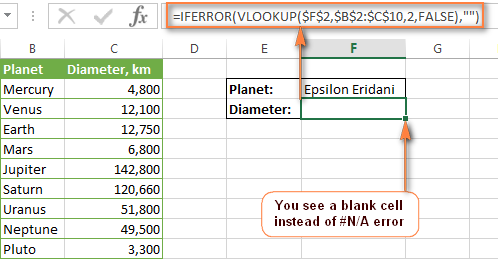If you'd rather display your own message instead of a standard Vlookup error, type it between the quotation marks, like this:

`=IFERROR(VLOOKUP(\$F\$2,\$B\$2:\$C\$10,2,FALSE),"Oops, no match is found. Please try again!")`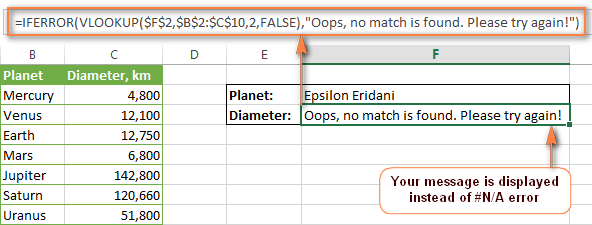#### Using VLOOKUP with ISERROR

Since the IFERROR function was introduced in Excel 2007 only, in lower Excel versions you will have to use the combination of IF and ISERROR functions in this way:

=IF(ISERROR(VLOOKUP formula), "Your message if any", VLOOKUP formula)

For example, here's the IF / ISERROR / VLOOKUP formula analogous to the IFERROR / VLOOKUP formula above:

`=IF(ISERROR(VLOOKUP(\$F\$2,\$B\$2:\$C\$10,2,FALSE)), "", VLOOKUP(\$F\$2,\$B\$2:\$C\$10,2,FALSE))`

That's all for today. Hopefully, this short tutorial will help you cope with all possible VLOOKUP errors and get your formulas to work in the way you want.

### 183 responses to "Excel VLOOKUP not working - solutions for N/A, NAME and VALUE errors"

1.Prakash KC says:

=IF(K9="one",IFERROR(VLOOKUP(N9,[One.xlsx]Ledger!\$A\$12:\$BI\$29,3,FALSE),"No Data"))&IF(K9="two",IFERROR(VLOOKUP(N9,[Two.xlsx]Ledger!\$A\$12:\$BI\$29,3,FALSE),"No Data"))&IF(K9="three",IFERROR(VLOOKUP(N9,[three.xlsx]Ledger!\$A\$12:\$BI\$29,3,FALSE),"No Data"))

Result FALSEMANISHA BISTAFALSE

how to remove FALSE

2.Badri says:

Hi,

I have problem with vlookup, if i perform the vlookup in the same file with different sheets, the formula is giving me partial results. However if i vlookup the same data from a different excel file, its giving me the complete results.

what would be the reason, Is there something i can do with the formula to work perfectly within sheets?

3.charles says:

I did a vlookup and the output showed this number (15359E+11) instead of the actual digits. I have not had this kind of error before. Please advise.

•VJ says:

Just convert it to NUMBER

4.Frank says:

The iferror function work greatly, the if(iserror way may still produce #VALUE! cells.

5.JO CHAWANDIT says:

THANK YOU. THIS IS QUITE USEFUL.

6.Irhan says:

Very Useful - keep up the good work ( share the knowledge..sharing is caring )

7.confuse says:

=VLOOKUP(IF(BC10>=130%,"130% & Above",IF(BC10<70%,"0.0 - 69%",ROUNDDOWN(BC10,2))),INDIRECT(BL10),2,0)
so the result should show rating
Sales Target RM25mil & Above
Rating Rating Range Ach % Rating Ach %
Outstanding 4.75-5.00 126% - Above 5.00 130% & Above
4.94 129%
4.88 128%
4.81 127%
4.75 126%
4.50-4.74 121% - 125% 4.74 125%
4.68 124%
4.62 123%
4.56 122%
4.50 121%

8.Narendra Kumawat says:

I have data in two different excel files. I tried to input VLOOKUP manually.

9.Doug Lee says:

Trying to do a vlookup on a table that is 1000 rows and 26 columns. If I try to get the value in the 13th column, it works fine. However, if I try for any column greater than that I get a #REF! error. All columns have valid data. Formula is =VLOOKUP(\$a1,TableSheet!\$A:\$Z,15,FALSE). Any ideas?

•Jeff Hayden says:

If you want to get work done, and run into excel misbehaving, go find an old copy of Lotus 1-2-3, install it, copy your data in and do your lookups there. The Lotus vlookup function isn't nearly as stupid or sensitive as excel, and doesn't require the lookup table data to be sorted just so to work properly.

10.Reena Sahu says:

excellent ,thank you

11.Kirill says:

Another possible issue: special characters in lookup value. E.g. tilde ~. Solution would be to duplicate this character: VLOOKUP(SUBSTITUTE(C1,"~","~~"),A:B,2,false)

12.Rayees Ahmed says:

i am using vlookup from another sheet, i have entered 3 entries that is ok but when i am doing next entry it pulls value from wrong row. please help me i have my file if you ask me i can mail you my file.

13.Amy says:

Hi, i use vlookup to match in the completion status from another file. but then some of my cell does not capture the formula and turn out the result is wrong. would anyone please help me out in this matter.

14.Will says:

When using vlookup, the formula only displays moot the value. No error messages. Any ideas?

15.Tony T. says:

Thanks for this outstanding reference, much appreciated! Also, good to know I'm not the only one that struggles with this in Excel.

16.Dina says:

Thank you for this, very helpful!

17.Lucy says:

I am using vlookup to copy values from one table to another. Many of the values have been copied, but not all. Those that have not been copied are showing #NV. I have checked for all the possible reasons for the error, corrected some, but the problem persists and has not changed at all. I even tried INDEX/MATCH with no avail. Any Idea what could be the cause?

18.Bonnie says:

When using vlookup, I can get the correct results for most of the table. When I get down to the last 4 lines of the table, it gives me results from previous lines when i change the lookup data. I have resorted the table multiple times but always get the same incorrect response.

19.Sheikh says:

20.dbp says:

VLOOKUP() will fail if, "the lookup column is not sorted in the ascending order." Well, that's just stupid.

21.Petr says:

hello,

can you help me_ please. This function =IFERROR(SVYHLEDAT(A3;'M (3)'!A3:I9988;3;1);"Not")
do not describe. Why?

Thanks

•Alexander Trifuntov (Ablebits.com Team) says:

Hello Petr!
I’m sorry but your task is not entirely clear to me. What is "SVYHLEDAT"? Could you please describe it in more detail? Thank you!

22.RAJAT AWASTHI says:

i am having a lookup related problem when i am applying lookup function to a cell which does not contain the actual data but there is formula applied to get the value from other two cell so now lookup is unable to look for that cell where formula has been filled but when i am typing actual value in that cell lookup function is working correctly but when there is a formula which is showing correct value lookup function is unable to work .
For example lookup can look for a value 3 and search it in an array but when there is two column containing 1 in first column and 2 in second column and now in third column i am using formula to add them simply so i sum them and now the value i am getting is 3 in third column but when i am applying lookup function to it. It is showing #N/A as usual but when instead of formula i am directly typing the value 3 in third column the lookup formula works perfectly so any solution on this will be helpful.

•Alexander Trifuntov (Ablebits.com Team) says:

Hello Rajat!
Most importantly, what function are you using for a search? Is it VLOOKUP or anything else? Please give me the formula which is performing a search.
What data are in the cells which that formula is referring to?

•GK says:

Hello Alexander,

I have a similar problem like Rajat. The 'lookup_value' reference in my Vlookup function is actually a cell with formula which is =RIGHT(A1,3). But vlookup is unable to read this and returning #N/A error, if I manually put the result of =RIGHT(A1,3) then vlookup is able to return the result. Also please note that if the result of the function =RIGHT(A1,3) is text then it work but unfortunately my result for this function is a number, so I guess this has something to do with formatting of the cell or something.

GK

•Alexander Trifuntov (Ablebits.com Team) says:

Hello!
Calculation result RIGHT(A1,3) - always text. If you need to search for a number, then I recommend using = VALUE(RIGHT(A1,3))
If you have any questions, don't hesitate to ask.

•GK says:

Hi

Working perfectly!!! Thank you so much!!!

GK

23.Maureen says:

Hi
How do I get the 0 to return as text in my formula, this leads on to pivot table and if it can find the value it presents as text but if it cant it presents as value so I get two lines in my pivot? =IFERROR(VLOOKUP(A2,'[Works in RAMM by month 19-20.xlsx]Sept to June 20'!\$A\$1:\$L\$276,12,FALSE),"0 ")
thanks Mo

•Alexander Trifuntov (Ablebits.com Team) says:

Hello Maureen!
If I understand your task correctly, maybe the following formula should work for you:

=IFERROR(VLOOKUP(A2,'[Works in RAMM by month 19-20.xlsx]Sept to June 20'!\$A\$1:\$L\$276,12,FALSE),"0" & " ")
Hope you’ll find this information helpful.

24.Pat says:

The numbers formatted as text gets me every time

•Alexander Trifuntov (Ablebits.com Team) says:

Hello Pat!
Please describe your problem in more detail. It’ll help me understand it better and find a solution for you. Thank you.

25.Moataz says:

That has really fixed my problem after long time searching, Thank you !!

26.Praveen L says:

Hi ,
While using Vlookup ,
Lookup value is a formula based reference created using the formula
"=TRIM(LEFT(A4,5)&MID(A4,10,3)&RIGHT(A4,4))" . A4 cell value is = 10211-40-000-0000.
after allpying the above formula i get value as "102110000000" in B4.
Now if i put vlookup or index match taking "B4 cell" as lookupvalue i m getting error as "#N/A" . However if paste special the same cell with values the formula works fine .
Regards
Praveen L

27.Bhagyesh says:

My formula was VLOOKUP(C2,Sheet2!A2:B45,2,FALSE). The expectation was that the output should be the value from column B on Sheet2 if there is a match. However, it never returned a value. Any ideas?
A similar VLOOKUP worked on the same sheet with no issues.

•Alexander Trifuntov (Ablebits.com Team) says:

Hello!
Your VLOOKUP formula has no errors. I don’t know what values you are looking for, but I suppose it is text. Perhaps there are extra spaces, non-printable characters in the text. I recommend checking for extra spaces and non-printing characters, as described in this article.

28.GL says:

I have a simple VLOOKUP table with unordered data. I have set up the formula properly (set to exact match, to lookup value in left col and return value in the right). Format is general for both the array and for the col of values being looked up (I don't have, for example, numbers with the apostrophe in front, indicating that they are text. The formula works for all values, except as follows:
ANY VALUE THAT CONTAINS PARENTHESES RETURNS AN #N/A, EVEN THOUGH THE IDENTICAL VALUE IS IN THE LEFT COL OF THE ARRAY. I have checked character counts, I have used EXACT in a sample case to confirm that the values in the array and in the col of values that I am looking up are identical—there are no extra spaces. I have deleted the parens and confirmed that I get a value, not an #N/A. I have replaced the parens with slashes—the formula works. I HAVE REPLACED THE PARENS WITH BRACKETS—THE FORMULA RETURNS AN #N/A.
Is this a known bug?

•Alexander Trifuntov (Ablebits.com Team) says:

Hello!
It’ll help me understand it better and find a solution for you. Thank you.

•GL says:

Thanks, Alexander! It's real simple—I am trying to confirm that a value in one col of my doc appears verbatim in another. Here is the set up:
Col A
value 1, 2, 3, etc. [different value in each row—the values are not ordered]
Col B
OK [I use the value "OK" in each row]
Col C
value 1, 2, 3, etc. [different value in each row—there cannot be a value in C that does not appear in A]
Col D
=VLOOKUP(C4;\$A\$1:\$B\$75;2;0) [this is the formula in row 4 of the doc—the first cell reference, Cx, always matches the given row]
I want to this formula in D to give me an #N/A if the value in C is not found in A, but an "OK" if the value in C appears in A. Very simple.
However, what I discovered is that, as soon as there are parentheses in the value, it doesn't matter if the value in C appears in A exactly as it appears in C—I get an #N/A. When I delete the parens in both A and C, I get my OK instead of the #N/A.

I am familiar with the problems created for EXACT, vLOOKUP, and other formulas by trailing space, by numbers converted to text, and so on. I am pretty confident that my problem is not due to these issues. Instead, I think the appearance of parentheses or brackets in the text causes vLOOKUP to fail. I was hoping folk could confirm—and perhaps supply a workaround. (Yes, I can replace the parens with another character before using vLOOKUP. Might there be something else to do in the formula?)

Thanks for any light you can shed!

•Alexander Trifuntov (Ablebits.com Team) says:

Hello!
The problem with parentheses in the VLOOKUP function is unknown to me. This did not cause problems for me. Maybe after the bracket there is still a space or some non-printable character? Try using the TRIM or CLEAR function:

= VLOOKUP(CLEAR(TRIM(C4));\$A\$1:\$B\$75;2;0)

I hope this will help, otherwise please do not hesitate to contact me anytime.

29.Victoria says:

The above guide still not solve my problem. I still
Hitting NA# in the VLOOKUP after I done edit or amend the cell format. Can I send u the file ? And you help to point out the issue ?

•Alexander Trifuntov (Ablebits.com Team) says:

Hello Victoria!
I’m sorry but your task is not entirely clear to me. For me to be able to help you better, please describe your task in more detail. Please specify what you were trying to find, what formula you used and what problem or error occurred. Give an example of the source data and the expected result.
It’ll help me understand it better and find a solution for you. Thank you.

30.Kasim says:

Thank you so much. Really appreciate it.

31.Blair says:

Hi. Really appreciate the write up. I am using excel for Mac. I used vlookup to match serial numbers. For the serial numbers, I have to use the tick mark ' to keep leading 0s. Vlookup is not matching not matter what I have tried. The odd thing is that if I double click in the first cell in the array table, the tick mark is removed and the match occurs. I am trying to avoid double clicking on thousands of cells to make vlookup work. Any ideas? Kind of urgent for a data conversion. THANKS!

•Handri says:

yeah me experience this weird error too. please any tips ASAP.

32.Ashok Reddy says:

one cell is having more than 100 values. so vlookup failed and getting NA but if use find it finding the value.

•Alexander Trifuntov (Ablebits.com Team) says:

Hello!
Clarify the question. One cell can only have 1 value

33.Griselda says:

I am having a format issue with vlookup.
If I copy from the vlookup data sheet like an id number it will work but if I type the id number it will return #NA.
- both data and input sheet have the column set as numbers
-tried copying and pasting the formatting from data to input sheet
Any help is appreciated :)

•Alexander Trifuntov (Ablebits.com Team) says:

Hello!
If your numbers are derived from calculations, I recommend using rounding. Your numbers may differ in decimal place, which is not shown on the screen.

34.Jason Gabler says:

ANOTHER POSSIBLE REASON VLOOKUP GET #N/A

If the lookup column has multiples of the same value it confuses lookup and will cause the #/NA error. In other words, the lookup value must have a unique presence in the lookup column.

•Svetlana Cheusheva (Ablebits.com Team) says:

Hi Jason,

In case of multiple matches, VLOOKUP returns the first found match. This may be confusing in many situations, so I agree that you'd better have unique values in the lookup column.

35.Muratcan says:

So my current issue is number with decimals. I got several sets of data with 123456.xyz. XYZ changes on each of them. I tried to vlookup with first 6 numbers, it wont detect if there is decimals involved. Any work around for that?

•Alexander Trifuntov (Ablebits.com Team) says:

Hello!
The VLOOKUP function searches for an exact match. If you are not using decimal numbers for your search, use the ROUND function to round your results.

•Muratcan says:

Thanks for the reply, tho in my case number after decimals important for me so rounding doesn't help. I was basically looking a way to sort the numbers based on values before decimals as they are fixed and values after decimals change. I guess VLOOKUP function can't help with that. Back to the drawing board!

•Alexander Trifuntov (Ablebits.com Team) says:

Hello!
You can round to 2 or 3 decimal places that are important to you.

36.Gus says:

Does anyone know why when typing a vlookup statement, the cell displays something like this =vlookup(A2,Table_Name,8,false). excel does not run the statement? just displays the characters of the statement. TIA

37.Krystal says:

Hi there,

Can someone help me with this formula for vlookup?

=VLOOKUP(P\$1:P\$32424,'Report 341.05'!A1:AM56914,17,FALSE) is not bringing me any data in the spreadsheet.

Thanks!

•Alexander Trifuntov (Ablebits.com Team) says:

Hello!
Without seeing your data it is difficult to give you any advice.
But Lookup_value cannot be a range (P\$1:P\$32424). Lookup_value can be a value (number, date or text), cell reference (reference to a cell containing a lookup value), or the value returned by some other function.

38.THENMOZHI says:

IN MY WORKSHEET BY MISTAKE I PRESSED SOME KEYS. IT SHOWS AS #VALUE! ... I TRIED IN MANY WAYS . BUT I COULDN'T GET IT AGAIN. ALL THE TEXT ENTRIES ONLY CHANGED AS #VALUE!. IN A WORKBOOK ALL THE 4 SHEET GOT THE SAME PROBLEM. GUIDE ME HOW TO SOLVE IT?

•Alexander Trifuntov (Ablebits.com Team) says:

Hello!

39.Zulqar nain says:

I applied vlookup from a sheet...the sum of result which I got through vlookup is greater than the sum in the original file ...how is this possible?

40.Martijn Bevelander says:

hi

I am using Vlookup on a very long list of "itemnumbers."
These "itemnumbers" consist of a combination of letters and numbers.
Some consist of numbers only.
Some consist of letters only.

All columns were set as text, prior to pasting the values.
Very often, Vlookup does not correctly find all of the "itemnumbers" which consist of numbers only.

0000123456789 vlookup does not find this
000123ABC0012 vlookup does find this
ABCDEABCDEXYZ vlookup does find this
ABC7890123456 vlookup does find this
8888885521111 vlookup does not find this

To fix this, i can go into each cell [F2] then hit [ENTER]
After that, vlookup does find a match.

Does anybody know of a better way to do this?

---
By the way,
The "extra spaces" problem, I usually "solve" by CTR+H … then replace empty spaces with nothing
That obviously works only when the data is not supposed to have any empty spaces
--

•Alexander Trifuntov (Ablebits.com Team) says:

Hello!
I could not repeat your mistakes. Your data may have come from another program. The data may contain extra spaces and non-printing characters. You can learn more about deleting spaces and non-printing characters in Excel in this article on our blog.

•Martijn Bevelander says:

hello Alexander

Thank you for trying.

yes, the data comes from different sources
1 huge list of itenmumbers from an ERP (with all sorts of info in other columns)
1 huge list of itemnumbers from another source (with different info in other columns)
I then use vlookup in excel to combine the data in 1 sheet.

To be able to do so, first i get all data to .dat or .txt to remove formats
Then I set a new empty excel sheet to text completely (not to general / not to number) this is very important.
Then I copy/paste values only to excel.

If there are any empty spaces, these are deleted with the CTR+H (find space and replace with nothing, works perfectly.)

To me, the resulting values from both sources look identical.
Excel does correctly match all of the text fields which contain a combination of letters and numbers (even if the first character is a zero 0)
Excel does correctly match all of the text fields which contain letters only.

However, excel does not correctly match the textfields which consist of numbers only.
By chance I found a labour intensive workaround
(after hitting ENTER while reviewing the data in a cell which should have been found by Vlookup.. suddenly the Vlookup did work for that 1 cell)

...so... I hit [F2] [ENTER] many, many times... and then it does work.

I was hoping somebody would know of a more efficient way.
Maybe there is some trick to mimic [F2] [ENTER] in all cells of a column.

•Alexander Trifuntov (Ablebits.com Team) says:

Hello!
Your data contains some kind of non-printable characters. I recommend using the article, the link to which I gave you earlier. I think this will help.